Next: Discussion Up: CCD Detectors Previous: CCD Detectors

## Introduction

The nominal output Xij of a CCD-element to a quantum of light Iijcan be given as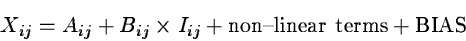(18.1)

This equation does not account for charge transfer inefficiencies and other effects known to exist in CCDs.

The dark current and the cold columns contribute to the additive term A; the quantum- and transfer-efficiency enter into the multiplicative term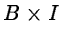. It is known that the response of the CCD is essentially linear so the non-linear terms are generally neglected. The Bias is normally added to the output electronically to avoid problems with digitising values near to zero.

The objective of the first step in reducing CCD-images is to determine the relative intensity Iij of a science data frame. In order to do this, two more frames are required in addition to the science picture, namely:

• FLAT-frames to determine the term Bij, and
• DARK-frames to describe the term Aij.
FLAT-fields are made by illuminating the CCD with a uniformly emitting source. The Flat-field then describes the sensitivity over the CCD which is not uniform. For FLAT-field exposures and SCIENCE-frames we get from Equation (B.1)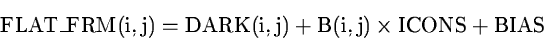(18.2)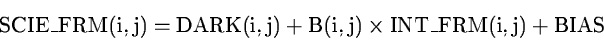(18.3)

where ICONS represents a flux from a uniform source, and BIAS is a constant signal which is added to the video signal of the CCD before being digitised.

The DARK-current is measured in the absence of any external input signal: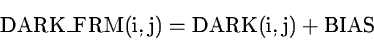(18.4)

Combining Eqs.(B.2), (B.3) and (B.4) we isolate: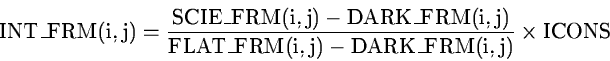(18.5)

ICONS can be any number. If set to the average signal of the dark-corrected FLAT-frame or a subimage thereof:(18.6)

then the reduced intensity frame INT_FRM will have similar data values as the original SCIE_FRM.Next: Discussion Up: CCD Detectors Previous: CCD Detectors
Petra Nass
1999-06-15